# Primary 6 PSLE Practice #2

Time elapsed:
Q1. Thaddeus has two \$5-notes and a \$1-note. How many 50-cent coins can he exchange them for?     [1 mark]
Q2. Which digit in 69.87 is in the tenths place?     [1 mark]
Q3. Simplify 6 + 9r - 2 + 2r     [1 mark]
Q4. Round off 87 935 to the nearest thousand.     [1 mark]
Q5. Mandy bought 1.4 m of ribbon to make 5 bows. What was the length of the ribbon used for each bow?     [1 mark]
Q6. A bank gives 2% interest per year. Xavier deposits \$2000 in the bank. How much interest will he receive at the end of one year?     [2 marks]
Q7. The graph below shows the number of members in a fitness club over a period of time. Which month did the fitness club have the greatest increase in the number of members?     [1 mark]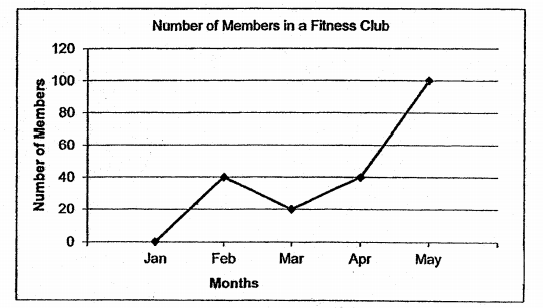Q8. The bar graph shows the number of visitors to a zoo from 2013 to 2017. During which one-year period was the increase in the number of visitors the greatest?     [1 mark]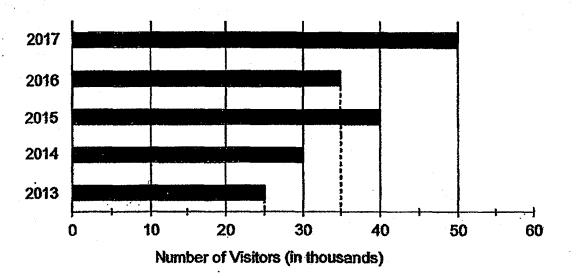Q9. Francis took 2h 15min to bake a cake. He started baking at 11.35am. What time did he finish baking?     [1 mark]
Q10. Which one of the following fractions is the smallest?     [1 mark]
Q11. In the figure below, ABC is an equilateral triangle. AE and BD are straight lines. Find ∠f.     [2 marks]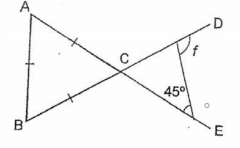Q12. The figure below, not drawn to scale, shows a circle that is divided into 4 parts, A, B, C, D. Line XY is the diameter of the circle. Area A is 12 of Area B. Area C is 5 times of Area D. The area of A is 26 cm2. Find the area of C.     [2 marks]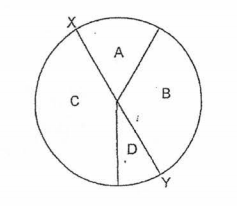Q13. A wheel of radius 14 cm made 10 complete turns. Find the distance covered. Take π = 227     [1 mark]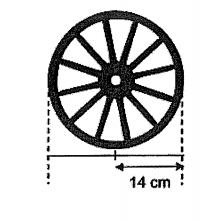Q14. The graph below shows the number of bowls of noodles sold at a stall over 5 days. How many bowls of noodles were sold from Monday to Wednesday altogether?     [1 mark]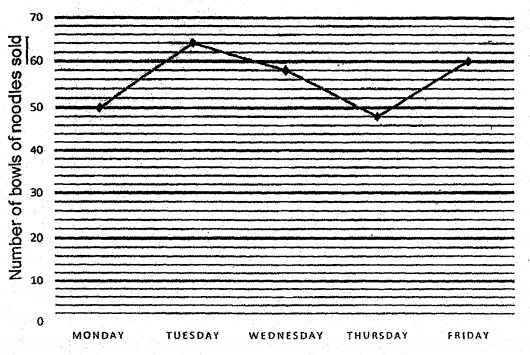Q15. The length and breadth of a rectangle are (4y + 5) cm and 2y cm respectively. What is the perimeter of the rectangle?     [1 mark]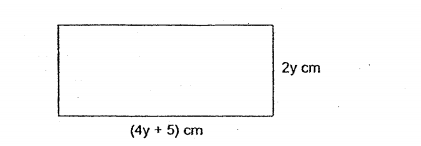Q16. A carpenter has a rectangular block of wood measuring 25 cm by 15 cm by 8 cm. What is the maximum number of 2-cm cubes that he can cut from it?     [1 mark]
Q17. Charlotte sold 120 cupcakes in the morning and 40% of the remaining cupcakes in the afternoon. The number of cupcakes left in the end was 13 of what she had at first. How many cupcakes were sold in the afternoon?     [2 marks]
Q18. What is the lowest common multiple of 6 and 8?     [1 mark]
Q19. Round 99 542 to the nearest thousand.     [1 mark]
Q20. Yvette left her home at 23 48. She took 20 minutes to reach the airport. What time did she reach the airport?     [1 mark]